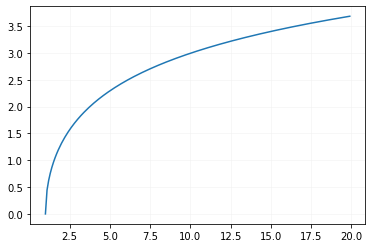# acosh()

acosh(x) returns inverse hyperbolic cosine of x , the input number x is in radian.
``````import math
print(math.acosh(1))       # 0.0
print(math.acosh(1.5))     # 0.9624236501192069
print(math.acosh(5))       # 2.2924316695611777``````
Note that all the inputs are in radian.
For any value less than 1 , we will get ValueError
``````import math
print(math.acosh(0.9))   ``````
Above code will generate error.

## Inputs in degree

We can convert radian value to degree and use the same
``````import math
in_degree = 60
print(math.acosh(in_redian)) # 0.30604210861326536``````
Output
``0.30604210861326536``

## Drawing graph of acosh()

We will use Matplotlib to generate graph of acosh``````import matplotlib.pyplot as plt
x=[]
y=[]
i=1
while (i<=20):
x.append(i)
y.append(math.acosh(i))
i=i+0.1
plt.plot(x,y)
plt.grid(linestyle='-',
linewidth=0.5,color='#f1f1f1')
plt.show()``````

Subscribe to our YouTube Channel here

## Subscribe

* indicates required
Subscribe to plus2netplus2net.com